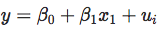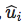Over a million developers have joined DZone.{{announcement.body}}
{{announcement.title}}

# Automatically Combining Factor Levels in R

DZone 's Guide to

# Automatically Combining Factor Levels in R

### A big data expert runs us through how to use the R language for some interesting data science problems, and how to visualize our results.

· Big Data Zone ·
Free Resource

Comment (0)

Save
{{ articles.views | formatCount}} Views

Each time we face real applications in an applied econometrics course, we have to deal with categorical variables. And the same question arises, from students: how can we automatically combine factor levels? Is there a simple R function?

I did upload a few blog posts, over the past few years. But so far, nothing satisfying. Let me write down a few lines about what could be done. And if someone wants to write a nice R function, that would be awesome. To illustrate the idea, consider the following (simulated dataset):

``````n=200
set.seed(1)
x1=runif(n)
x2=runif(n)
y=1+2*x1-x2+rnorm(n,0,.2)
LB=sample(LETTERS[1:10])
b=data.frame(y=y,x1=x1,
x2=cut(x2,breaks=
c(-1,.05,.1,.2,.35,.4,.55,.65,.8,.9,2),
labels=LB))
str(b)
'data.frame':200 obs. of  3 variables:
\$ y : num  1.345 1.863 1.946 2.481 0.765 ...
\$ x1: num  0.266 0.372 0.573 0.908 0.202 ...
\$ x2: Factor w/ 10 levels "I","A","H","F",..: 4 4 6 4 3 6 7 3 4 8 ...
table(b\$x2)[LETTERS[1:10]]

A  B  C  D  E  F  G  H  I  J
11 12 23 34 23 36 12 32  3 14``````

There is one (continuous) dependent variable y, one continuous covariable x_1, and one categorical variable x_2, with ten levels. We can plot the data using:

``````plot(b\$x1,y,col="white",xlim=c(0,1.1))
text(b\$x1,y,as.character(b\$x2),cex=.5)``````The output of a linear regression yield the following predictions:``````for(i in 1:10){
p=function(x) predict(lm(y~x1+x2,data=b),newdata=data.frame(x1=x,x2=LETTERS[i]))
u=seq(-1,1.065,by=.01)
v=Vectorize(p)(u)
lines(u,v)}``````

The slope for x_1 is the same, we simply add a different constant for each level. As we can see, some levels are very very close, so it seems legitimate to combine them into one single category. Here is the output of the linear regression:

``````summary(lm(y~x1+x2,data=b))
Coefficients:
Estimate Std. Error t value Pr(&gt;|t|)
(Intercept)  0.843802   0.119655   7.052 3.23e-11 ***
x1           1.992878   0.053838  37.016  &lt; 2e-16 ***
x2A          0.055500   0.131173   0.423   0.6727
x2H          0.009293   0.121626   0.076   0.9392
x2F         -0.177002   0.121020  -1.463   0.1452
x2B         -0.218152   0.130192  -1.676   0.0955 .
x2D         -0.206970   0.121294  -1.706   0.0896 .
x2G         -0.407417   0.129999  -3.134   0.0020 **
x2C         -0.526708   0.123690  -4.258 3.24e-05 ***
x2J         -0.664281   0.128126  -5.185 5.54e-07 ***
x2E         -0.816454   0.123625  -6.604 3.94e-10 ***
---
Signif. codes:  0 ‘***’ 0.001 ‘**’ 0.01 ‘*’ 0.05 ‘.’ 0.1 ‘ ’ 1

Residual standard error: 0.2014 on 189 degrees of freedom
Multiple R-squared:  0.8995,Adjusted R-squared:  0.8942
F-statistic: 169.1 on 10 and 189 DF,  p-value: &lt; 2.2e-16
AIC(lm(y~x1+x2,data=b))
 -60.74443
BIC(lm(y~x1+x2,data=b))
 -21.16463``````

Here the reference category is "I". And it looks like we could actually combine that category with several others. One strategy here would be to select all categories that seem to be not significantly different, and to run a (multiple) test:

``````library(car)
linearHypothesis(lm(y~x1+x2,data=b), c("x2A = 0", "x2H = 0", "x2F = 0"))

Hypothesis:
x2A = 0
x2H = 0
x2F = 0

Model 1: restricted model
Model 2: y ~ x1 + x2

Res.Df    RSS Df Sum of Sq      F Pr(&gt;F)
1    192 8.4651
2    189 7.6654  3   0.79971 6.5726  3e-04 ***
---
Signif. codes:  0 ‘***’ 0.001 ‘**’ 0.01 ‘*’ 0.05 ‘.’ 0.1 ‘ ’ 1``````

It seems that we can combine those four categories together.

Here, we can see what's going on when we change the reference category (actually, loop on all categories):

``````P=matrix(NA,nlevels(b\$x2),nlevels(b\$x2))
colnames(P)=rownames(P)=LETTERS[1:10]
plot(1:nlevels(b\$x2),1:nlevels(b\$x2),col="white",xlab="",ylab="",axes=F,xlim=c(0,10.5),
ylim=c(0,10.5))
text(1:10,0,LETTERS[1:10])
text(0,1:10,LETTERS[1:10])
for(i in 1:nlevels(b\$x2)){
#levels(b\$x2)=LETTERS[1:10]
b\$x2=relevel(b\$x2,LETTERS[i])
p=summary(lm(y~x1+x2,data=b))\$coefficients[-(1:2),4]
names(p)=substr(names(p),3,3)
P[LETTERS[i],names(p)]=p
p=P[LETTERS[i],]
idx=which(p&gt;.05)
points(((1:10))[idx],rep(i,length(idx)),pch=1,cex=2)
idx=which(p&gt;.1)
points(((1:10))[idx],rep(i,length(idx)),pch=19,cex=2)}``````We are glad to see that it is symmetric: if "H" should be combined with "I", "I" should also be combined with "H".

Here, black points are related with the 10% p-value, and white points the 5% p-value. This graph is actually hard to read... And actually, this reminds us of Bertin (1967).Here, we can manually predefine some ordering (we will see below how it might be automatized):

``````LETTERSord=c("I","A","H","F","B","D","G","C","J","E")
P=matrix(NA,nlevels(b\$x2),nlevels(b\$x2))
colnames(P)=rownames(P)=LETTERSord
plot(1:nlevels(b\$x2),1:nlevels(b\$x2),col="white",xlab="",ylab="",axes=F,xlim=c(0,10.5),
ylim=c(0,10.5))
ct=c(3,3,2,1,1)
abline(v=.5+c(0,cumsum(ct)),lty=2)
abline(h=.5+c(0,cumsum(ct)),lty=2)
text(1:10,0,LETTERSord)
text(0,1:10,LETTERSord)
for(i in 1:nlevels(b\$x2)){
#levels(b\$x2)=LETTERS[1:10]
b\$x2=relevel(b\$x2,LETTERSord[i])
p=summary(lm(y~x1+x2,data=b))\$coefficients[-(1:2),4]
names(p)=substr(names(p),3,3)
P[LETTERSord[i],names(p)]=p
p=P[LETTERSord[i],]
idx=which(p&gt;.05)
points(((1:10))[idx],rep(i,length(idx)),pch=1,cex=2)
idx=which(p&gt;.1)
points(((1:10))[idx],rep(i,length(idx)),pch=19,cex=2)
}``````

Here we get the following:It looks like we have our combined categories...

Actually, it is possible to use another strategy. We start from some level, say "A". Then, we merge it with all non-significantly different levels. If "B" is not one of them, we use it as the new reference. Etc.

``````for(i in 1:nlevels(b\$x2)){
if(LETTERS[i]%in%levels(b\$x2)){
b\$x2=relevel(b\$x2,LETTERS[i])
p=summary(lm(y~x1+x2,data=b))\$coefficients[-(1:2),4]
names(p)=substr(names(p),3,nchar(p))
idx=which(p&gt;.05)
mix=c(LETTERS[i],names(p)[idx])
b\$x2=recode(b\$x2, paste("c('",paste(mix,collapse = "','"),"')='",paste(mix,collapse = "+"),"'",sep=""))
}}``````

The final categories are:

``````table(b\$x2)

A+I+H B+D+F   C+G     E     J
46    82    35    23    14``````

With the following regression output:

``````summary(lm(y~x1+x2,data=b))

Coefficients:
Estimate Std. Error t value Pr(&gt;|t|)
(Intercept)  0.86407    0.03950  21.877  &lt; 2e-16 ***
x1           1.99180    0.05323  37.417  &lt; 2e-16 ***
x2B+D+F     -0.21517    0.03699  -5.817 2.44e-08 ***
x2C+G       -0.50545    0.04528 -11.164  &lt; 2e-16 ***
x2E         -0.83617    0.05128 -16.305  &lt; 2e-16 ***
x2J         -0.68398    0.06131 -11.156  &lt; 2e-16 ***
---
Signif. codes:  0 ‘***’ 0.001 ‘**’ 0.01 ‘*’ 0.05 ‘.’ 0.1 ‘ ’ 1

Residual standard error: 0.2008 on 194 degrees of freedom
Multiple R-squared:  0.8975,Adjusted R-squared:  0.8948
F-statistic: 339.6 on 5 and 194 DF,  p-value: &lt; 2.2e-16
AIC(lm(y~x1+x2,data=b))
 -66.76939
BIC(lm(y~x1+x2,data=b))
 -43.68117``````

Which is consistent with the group we got before. But actually, if we change the order, we can get different combinations. For instance, if we go from "J" to "A", instead of "A" to "J", we obtain:

``````for(i in nlevels(b\$x2):1){
#levels(b\$x2)=LETTERS[1:10]
if(LETTERS[i]%in%levels(b\$x2)){
b\$x2=relevel(b\$x2,LETTERS[i])
p=summary(lm(y~x1+x2,data=b))\$coefficients[-(1:2),4]
names(p)=substr(names(p),3,nchar(p))
idx=which(p&gt;.05)
mix=c(LETTERS[i],names(p)[idx])
b\$x2=recode(b\$x2, paste("c('",paste(mix,collapse = "','"),"')='",paste(mix,collapse = "+"),"'",sep=""))
}}
table(b\$x2)

E         G+C I+A+B+D+F+H           J
23          35         128          14``````

with different information criteria here:

``````AIC(lm(y~x1+x2,data=b))
 -36.61665
BIC(lm(y~x1+x2,data=b))
 -16.82675``````

I guess it would be necessary to randomly run the order in which we go through the levels. Last, but not least, one can use regression trees. The problem is that there is another explanatory variable that might interphere. So I would suggest (1) to fit a linear modelto calculate the residuals,(2) to run a regression tree, to explainwith categorical variable x_2 (I did explain how trees are build when the explanatory variable is a categorical one in a previous post):

``````library(rpart)
library(rpart.plot)
b\$e=residuals(lm(y~x1,data=b))
arbre=rpart(e~x2,data=b)
prp(arbre,type=2,extra=1)``````Observe that the leaves have the same groups as the one we got.

``````arbre
n= 200

node), split, n, deviance, yval
* denotes terminal node

1) root 200 22.563500  7.771561e-18
2) x2=G,C,J,E 72  4.441495 -3.232525e-01
4) x2=J,E 37  1.553520 -4.578492e-01 *
5) x2=G,C 35  1.509068 -1.809646e-01 *
3) x2=I,A,H,F,B,D 128  6.366628  1.818295e-01
6) x2=F,B,D 82  2.983381  1.048246e-01 *
7) x2=I,A,H 46  2.030229  3.190993e-01 *``````

I guess that it should be possible to put all that in an R function, to suggest combinations of level that might improve the regression.

Topics:
big data ,r ,data visualization ,data science ,tutorial

Comment (0)

Save
{{ articles.views | formatCount}} Views

Published at DZone with permission of

Opinions expressed by DZone contributors are their own.

# {{ parent.title || parent.header.title}}

{{ parent.tldr }}

{{ parent.urlSource.name }}